# Speed of clock

In how many minutes will the hour hand pass a straight angle and a right angle?

a =  360 min
b =  180 min

### Step-by-step explanation: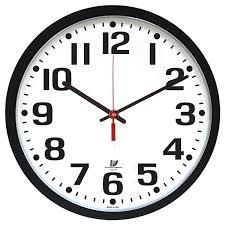Did you find an error or inaccuracy? Feel free to write us. Thank you!Tips to related online calculators
Do you want to convert velocity (speed) units?
Do you want to convert time units like minutes to seconds?

## Related math problems and questions:

• Time clockWhat are the angle of the hour hand and the minute hand if it is 0:40?
• HandsThe clock shows 12 hours. After how many minutes will angle between the hour and minute hand 90°? Consider the continuous movement of both hands hours.
• Little hand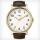What angle shifted little hand on the clock after one hour and 38 minutes?
• Minute handWhat is the distance the minute hand of the clock travels in 12 minutes, if the diameter of the clock is 30 cm and the hand extends to a distance of 2 cm from the edge of the clock?
• ClocksHow will long path walk the end of the clock's minute-hand long 7 cm for 330 minutes? How long will walk in the same time hour-hand?
• Minute-hand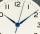How long distance will travel a large (minute) hand on the clock for 48 minutes, if its length is 56 cm?
• LunchLunch is given to seniors from 12:15 to 12:40 during the Coronavirus pandemic. What angle will the minute hand of clock describe during this time?
• Clock hands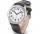The hands-on clock shows the time 12 hours and 2 minutes. Calculate the size of an acute angle between clock hands three hours later.
• Clock Tower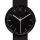What angle is betwenn hands on Clock Tower when show 17 hours and 35 minutes?
• Clock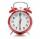What distance will pass end of 8 cm long hour hand for 15 minutes?
• Minute hand v2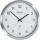In how many minutes describe the minute hand angle 60 degrees?
• Tram lines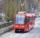Trams of five lines driven at intervals of 5,8,10,12 and 15 minutes. At 12 o'clock come out of the station at the same time. About how many hours again all meet? How many times have earch tram pass for this stop?
• Quarter of an hourIf the clock showed a quarter of an hour more, it would be 10 minutes to 10 hours. How many hours do they show?
• The tourist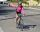The tourist came started from the hostel at an average speed of 5km/h. Half an hour later, the bicyclist started along the same route at a speed of 20km/h. How many minutes will a cyclist catch up and how many kilometers will he go?
• Car loop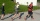The toy car runs at an average speed of 2 m/s. In a minute, it will pass the entire circuit five times. How long is the loop?
• Constant motionThe car has undergone constant motion 120 km in 1 hour 30 minutes. Determine its average speed. Determine how long the car drove in the first 20 minutes and 50 minutes from the start of the move.
• Motion againstFrom two places 36 km apart, a pedestrian and a cyclist will go straight opposite each other. How long will they meet if the speed of the pedestrian is 4 km/h and the cyclist 12 km/h?